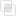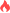python参数调用的注意点

python参数调用2021-05-27 17:09:50725浏览 · 0收藏 · 0评论1、数量定义时必须一致

2、位置必须要与定义时位置一致

3、实例

```x=y=z=0
def num(x,y,z):
x+=x
y+=y
z+=z
print(x,y,z)
num(x=1,y=2,z=3)
print(x,y,z)```python参数类型错误是什么如何规避python参数的初始化次数？设置python参数遵守优先级Python参数类型有几种？python参数中默认值的执行python参数解包的实现python参数是什么python参数默认值如何使用java局部变量表的介绍262

php中__sleep方法的使用708

python中Pylint的信息类型359